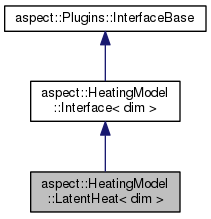ASPECT
aspect::HeatingModel::LatentHeat< dim > Class Template Reference
Inheritance diagram for aspect::HeatingModel::LatentHeat< dim >:[legend]

## Public Member Functions

void evaluate (const MaterialModel::MaterialModelInputs< dim > &material_model_inputs, const MaterialModel::MaterialModelOutputs< dim > &material_model_outputs, HeatingModel::HeatingModelOutputs &heating_model_outputs) const overridePublic Member Functions inherited from aspect::HeatingModel::Interface< dim >
virtual ~Interface ()=default

virtual void initialize ()

virtual void update ()

virtual void parse_parameters (ParameterHandler &prm)

virtual void create_additional_material_model_outputs (MaterialModel::MaterialModelOutputs< dim > &outputs) const

virtual void create_additional_material_model_inputs (MaterialModel::MaterialModelInputs< dim > &inputs) constStatic Public Member Functions inherited from aspect::HeatingModel::Interface< dim >
static void declare_parameters (ParameterHandler &prm)

## Detailed Description

### template<int dim> class aspect::HeatingModel::LatentHeat< dim >

A class that implements a standard formulation of latent heat. This includes a left hand side and a right hand side term:

The left hand side is $$-\rho T \frac{\partial S}{\partial T} \frac{D T}{D t}$$ so that we can add $$-\rho T \frac{\partial S}{\partial T}$$ to the $$\rho C_p$$ term.

The right-hand side term from latent heating is $$\frac{\partial S}{\partial p} T \rho (u \dot \nabla p)$$.

T, u, and p are the solutions from the previous time step or are extrapolated from there, depending on what is provided in the input arguments of this function.

Formulation modified after Christensen, Ulrich R. & Yuen, David A.: Layered convection induced by phase transitions, Journal of Geophysical Research: Solid Earth (1985).

Also see the Equations section in the manual.

Definition at line 59 of file latent_heat.h.

## § evaluate()

template<int dim>
 void aspect::HeatingModel::LatentHeat< dim >::evaluate ( const MaterialModel::MaterialModelInputs< dim > & material_model_inputs, const MaterialModel::MaterialModelOutputs< dim > & material_model_outputs, HeatingModel::HeatingModelOutputs & heating_model_outputs ) const
overridevirtual

Compute the heating model outputs for this class.

Implements aspect::HeatingModel::Interface< dim >.

The documentation for this class was generated from the following file: